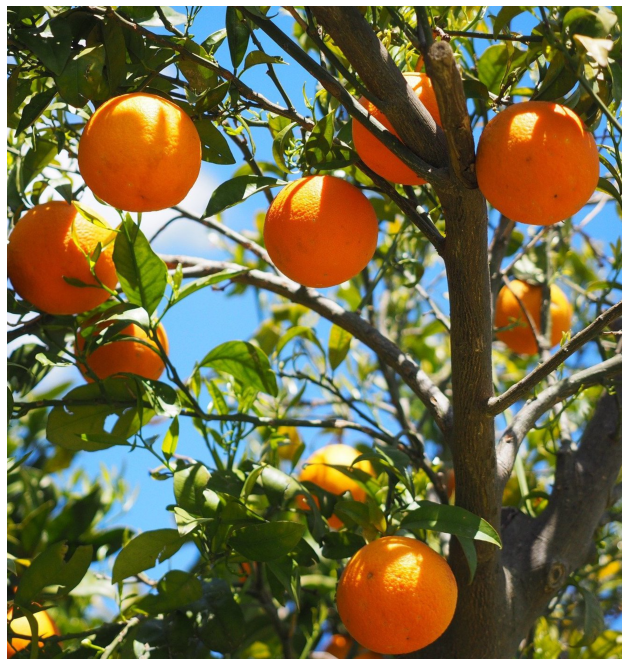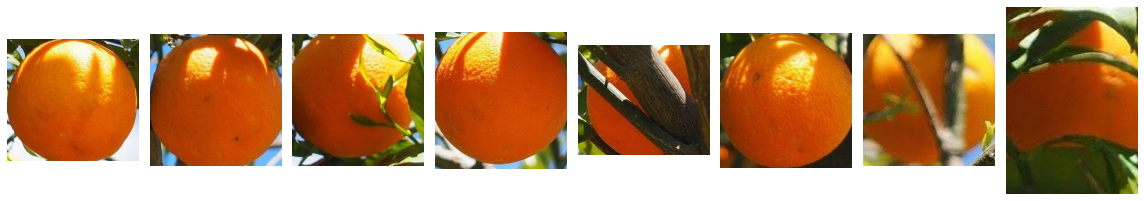### Categories

This post explains how to implement a function to generate a crop of a particular object from an image given the position of the centroid of the object. The images below show an example use case: cropping all the individual oranges from an image.This example uses the image of oranges from the NMS and IoU posts.

!curl -o oranges.jpg https://lh3.googleusercontent.com/pw/ACtC-3fLFL_y58xx1GVy6jLQ0quLpoctt-WG5yo5dR1N3RurI4Qodnnj_JeCEQG-kzILCAUNgZmcA5QlkuLYnbW33Y1XTj48knehvFywJoz1ni3U6MtGiiJzvz4edv0kU0y7RzYRvuWXbewA5glVbkx_Ja-PXg=w1312-h1393-no


### Define the function

Torchvision has several image transforms such as CenterCrop, RandomResizedCrop, FiveCrop etc but if you want to generate a custom crop from an image, there is a utils function called crop that takes as input the position of the top left corner of the crop region, along with the height and width of the crop.ped image If the crop is smaller than the required input size, then the image is padded with zeros.

In this example, the position of the centroid of the cropped region is known. Below, we define a wrapper that uses the torchvision crop function.

import math
import numpy as np
import matplotlib.pyplot as plt
import torch
from torchvision.transforms.functional import crop

def generate_crop(
image: torch.Tensor,
centroid_x: int,
centroid_y: int,
crop_width: int = 256,
crop_height: int = 256,
) -> torch.Tensor:
"""
Generate a crop of an image around a central point
with a specified width and height.

Args:
image: FloatTensor of shape (H, W, 3)
centroid_x: int, giving the x position
of the centre of the crop area
centroid_y: int, giving the y position
of the centre of the crop area
crop_width: int, width of the cropped image
crop_height: int height of the cropped image
Returns:
cropped_image: FloatTensor of shape
(crop_height, crop_width, 3)
"""
# Find the top and left positions of the crop
# region in the input image
left = math.floor(centroid_x - crop_width/2)
top = math.floor(centroid_y - crop_height/2)

# Reshape image to (3, H, W)
image = image.permute(2, 0, 1)

# Generate the crop
cropped_image = crop(image, top, left, crop_height, crop_width)

# Reshape back to (H, W, 3)
return cropped_image.permute(1, 2, 0)


### Example use case

Now we can apply this function to generate crops of all the oranges in the input image

img = plt.imread('oranges.jpg')
image_tensor = torch.from_numpy(img)

# The boxes are defined as [center_x, center_y, width, height]
boxes =  [
[285, 310, 268, 248],
[1130, 330, 258, 258],
[135, 550, 250, 250],
[670, 490, 230, 240],
[840, 265, 240, 200],
[830, 1258, 245, 250],
[1120, 670, 150, 150],
[245, 750, 120, 170]
]

# Generate crop for each orange
crops = [generate_crop(image_tensor, *box) for box in boxes]

# Visualize each cropped image
fig, axs = plt.subplots(nrows=2, ncols=4, figsize=(16, 16), )
axs = np.array(axs).reshape(-1)

for ax, crop_im in zip(axs, crops):
ax.imshow(crop_im)
plt.axis('off')

plt.tight_layout()